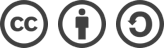## SDUT 3554 无尽走廊（动态规划）解题报告

2016-06-08 1,075 次浏览

## 题面

Time Limit: 1000ms Memory limit: 65536K

1代表正面，0代表反面。

4
1 0 0 1

4

## 解题思路

int max_sum = 0, this_sum = 0;
for(int i=0; i<n; ++i) {
this_sum += a[i]; //向右累加
if(this_sum > max_sum) //更新最大子序列和
max_sum = this_sum;
if(this_sum < 0) //如果当前子序列和为负
this_sum = 0; //则抛弃当前子序列
}

#include <cstdio>
using namespace std;

int main(int argc, char const *argv[]) {
int n, a;
while(~ scanf("%d", &n)) {
//sum 为初始状态的正面向上的硬币数
int sum = 0;
for(int i=0; i<n; ++i) {
scanf("%d", &a[i]);
if(a[i] == 1) sum++;
}
int max_sum = 0, this_sum = 0;
for(int i=0; i<n; ++i) {
if(a[i] == 0) //反面翻到正面看作 1
this_sum++;
else //正面翻到反面看作 -1
this_sum--;
if(this_sum > max_sum)
max_sum = this_sum;
if(this_sum < 0)
this_sum = 0;
}
printf("%d\n", sum + max_sum);
}

return 0;
}bLue 创作，采用 知识共享署名 3.0，可自由转载、引用，但需署名作者且注明文章出处。